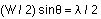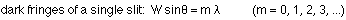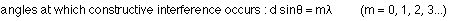## Dispersion, Diffraction and Diffraction Gratings

8-1-00

Sections 24.4 - 24.7

### Dispersion

Although we talk about an index of refraction for a particular material, that is really an average value. The index of refraction actually depends on the frequency of light (or, equivalently, the wavelength). For visible light, light of different colors means light of different wavelength. Red light has a wavelength of about 700 nm, while violet, at the other end of the visible spectrum, has a wavelength of about 400 nm.

This doesn't mean that all violet light is at 400 nm. There are different shades of violet, so violet light actually covers a range of wavelengths near 400 nm. Likewise, all the different shades of red light cover a range near 700 nm.

Because the refractive index depends on the wavelength, light of different colors (i.e., wavelengths) travels at different speeds in a particular material, so they will be refracted through slightly different angles inside the material. This is called dispersion, because light is dispersed into colors by the material.

When you see a rainbow in the sky, you're seeing something produced by dispersion and internal reflection of light in water droplets in the atmosphere. Light from the sun enters a spherical raindrop, and the different colors are refracted at different angles, reflected off the back of the drop, and then bent again when they emerge from the drop. The different colors, which were all combined in white light, are now dispersed and travel in slightly different directions. You see red light coming from water droplets higher in the sky than violet light. The other colors are found between these, making a rainbow.

Rainbows are usually seen as half circles. If you were in a plane or on a very tall building or mountain, however, you could see a complete circle. In double rainbows the second, dimmer, band, which is higher in the sky than the first, comes from light reflected twice inside a raindrop. This reverses the order of the colors in the second band.

### Diffraction

When we talked about sound waves we learned that diffraction is the bending of waves that occurs when a wave passes through a single narrow opening. The analysis of the resulting diffraction pattern from a single slit is similar to what we did for the double slit. With the double slit, each slit acted as an emitter of waves, and these waves interfered with each other. For the single slit, each part of the slit can be thought of as an emitter of waves, and all these waves interfere to produce the interference pattern we call the diffraction pattern.

After we do the analysis, we'll find that the equation that gives the angles at which fringes appear for a single slit is very similar to the one for the double slit, one obvious difference being that the slit width (W) is used in place of d, the distance between slits. A big difference between the single and double slits, however, is that the equation that gives the bright fringes for the double slit gives dark fringes for the single slit.

To see why this is, consider the diagram below, showing light going away from the slit in one particular direction.In the diagram above, let's say that the light leaving the edge of the slit (ray 1) arrives at the screen half a wavelength out of phase with the light leaving the middle of the slit (ray 5). These two rays would interfere destructively, as would rays 2 and 6, 3 and 7, and 4 and 8. In other words, the light from one half of the opening cancels out the light from the other half. The rays are half a wavelength out of phase because of the extra path length traveled by one ray; in this case that extra distance is :The factors of 2 cancel, leaving:The argument can be extended to show that :The bright fringes fall between the dark ones, with the central bright fringe being twice as wide, and considerably brighter, than the rest.

### Diffraction effects with a double slit

Note that diffraction can be observed in a double-slit interference pattern. Essentially, this is because each slit emits a diffraction pattern, and the diffraction patterns interfere with each other. The shape of the diffraction pattern is determined by the width (W) of the slits, while the shape of the interference pattern is determined by d, the distance between the slits. If W is much larger than d, the pattern will be dominated by interference effects; if W and d are about the same size the two effects will contribute equally to the fringe pattern. Generally what you see is a fringe pattern that has missing interference fringes; these fall at places where dark fringes occur in the diffraction pattern.

### Diffraction gratings

We've talked about what happens when light encounters a single slit (diffraction) and what happens when light hits a double slit (interference); what happens when light encounters an entire array of identical, equally-spaced slits? Such an array is known as a diffraction grating. The name is a bit misleading, because the structure in the pattern observed is dominated by interference effects.

With a double slit, the interference pattern is made up of wide peaks where constructive interference takes place. As more slits are added, the peaks in the pattern become sharper and narrower. With a large number of slits, the peaks are very sharp. The positions of the peaks, which come from the constructive interference between light coming from each slit, are found at the same angles as the peaks for the double slit; only the sharpness is affected.Why is the pattern much sharper? In the double slit, between each peak of constructive interference is a single location where destructive interference takes place. Between the central peak (m = 0) and the next one (m = 1), there is a place where one wave travels 1/2 a wavelength further than the other, and that's where destructive interference takes place. For three slits, however, there are two places where destructive interference takes place. One is located at the point where the path lengths differ by 1/3 of a wavelength, while the other is at the place where the path lengths differ by 2/3 of a wavelength. For 4 slits, there are three places, for 5 slits there are four places, etc. Completely constructive interference, however, takes place only when the path lengths differ by an integral number of wavelengths. For a diffraction grating, then, with a large number of slits, the pattern is sharp because of all the destructive interference taking place between the bright peaks where constructive interference takes place.

Diffraction gratings, like prisms, disperse white light into individual colors. If the grating spacing (d, the distance between slits) is known and careful measurements are made of the angles at which light of a particular color occurs in the interference pattern, the wavelength of the light can be calculated.